# 数据结构与算法学习笔记

#### 数据结构与算法思维导图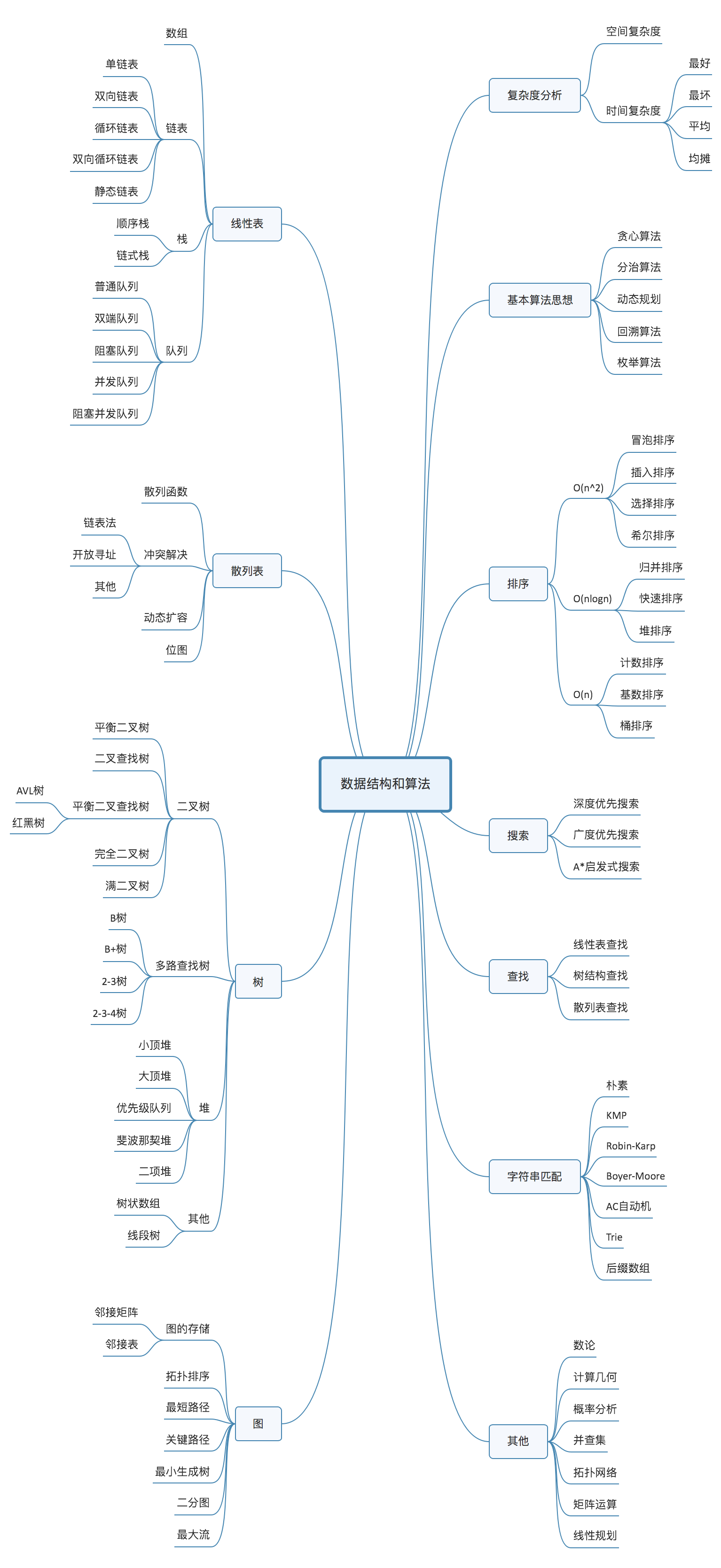• 数据结构：数组、链表、栈、队列、散列表、二叉树、堆、跳表、图、Tire树
• 算法： 递归、排序、二分查找、搜索、哈希算法、贪心算法、分治算法、回溯算法、动态规划、字符串匹配算法

## 1  算法的复杂度

#### 1.1大O复杂度表示法

公式:T(n)表示代码执行的时间; n表示数据规模的大小; f(n) 表示每行代码执行的次数总和。因为这是一个公式， 所以用f(n)来表示。公式中的O,表示代码的执行时间T(n)与f(n)表达式成正比。

所以，第一个例子中的T(n) = O(2n+2)，第二个例子中的T(m) = 0(2n2 +2n+3)。这就是大O时间复杂度表示法。大O时间复杂度实际上并不具体表示代码真正的执行时间，而是表示代码执行时间随数据规模增长的变化趋势，所以，也叫作渐进时间复杂度(asymptotic time complexity)，简称时间复杂度。

当n很大时，你可以把它想象成10000、100000。 而公式中的低阶、常量、系数三部分并不左右增长趋势,所以都可以忽略。我们只需要记录一个最大量级就可以了，如果用大O表示法表示刚讲的那两段代码的时间复杂度,就可以记为: T(n) = O(n); T(n)= 0(n2)。

#### 1.2.复杂度分析法则

1）单段代码看高频：比如循环。
2）多段代码取最大：比如一段代码中有单循环和多重循环，那么取多重循环的复杂度。
3）嵌套代码求乘积：比如递归、多重循环等
4）多个规模求加法：比如方法有两个参数控制两个循环的次数，那么这时就取二者复杂度相加。

1.3 时间复杂度分析

• 只关注循环执行次数最多的一段代码
• 加法法则：总复杂度等于量级最大的那段代码的复杂度
• 乘法法则：嵌套代码的复杂度等于嵌套内外代码复杂度的乘积

1.4 几种常见时间复杂度实例分析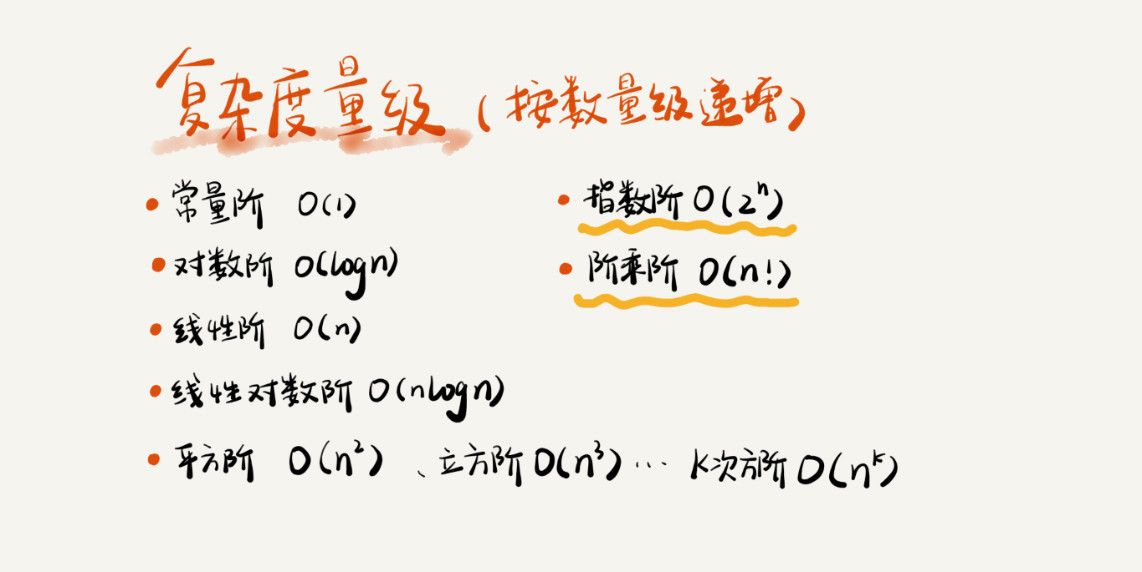O(1)（常数阶）、O(logn)（对数阶）、O(n)（线性阶）、O(nlogn)（线性对数阶）、O(n^2)（平方阶）、O(n^3)（立方阶）

O(2^n)（指数阶）、O(n!)（阶乘阶）

• O(1) :

• O(logn)、O(nlogn)
i=1;
while(i<=n) {
i = i*2;
}x=log2n，所以，这段代码的时间复杂度就是 O(log2n)

• O(m+n)、O(m*n)

   int cal(int m, int n) {
int sum_1=0;
int i=1;
for(;i<m;++i){
sum_1 = sum_1 + i;
}
int sum_2 = 0;
int j=1;
for (;j<n;++j){
sum_2 = sum_2 + j;
}
return sum_1 + sum_2;
}

1.5 空间复杂度分析

void print(int n) {
inti=0;
int[] a = new int[n];
for (i; i <n; ++i) {
a[i] =i* i;
}
for(i=n-1;i>=0;--i){
print out a[i]
}
}

1.6 复杂度增长趋势图: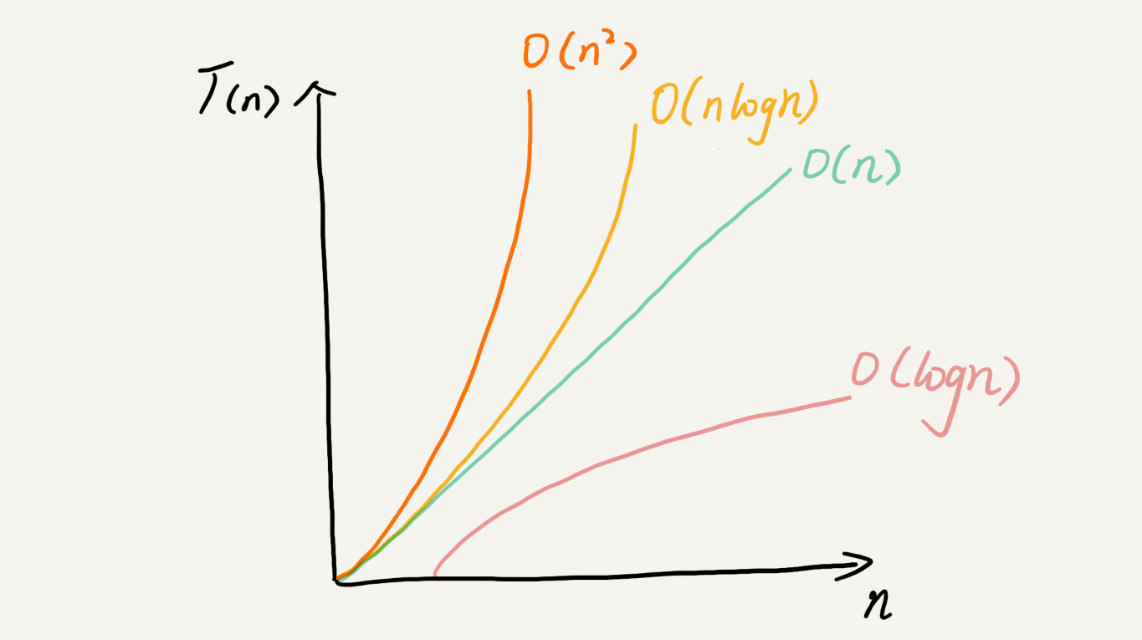1.最坏情况时间复杂度：代码在最坏情况下执行的时间复杂度。
2.最好情况时间复杂度：代码在最理想情况下执行的时间复杂度。
3.平均时间复杂度：代码在所有情况下执行的次数的加权平均值。
4.均摊时间复杂度：在代码执行的所有复杂度情况中绝大部分是低级别的复杂度，个别情况是高级别复杂度且发生具有时序关系时，可以将个别高级别复杂度均摊到低级别复杂度上。基本上均摊结果就等于低级别复杂度。

1.同一段代码在不同情况下时间复杂度会出现量级差异，为了更全面，更准确的描述代码的时间复杂度，所以引入这4个概念。
2.代码复杂度在不同情况下出现量级差别时才需要区别这四种复杂度。大多数情况下，是不需要区别分析它们的。

1.平均时间复杂度

2.均摊时间复杂度

### 1、数组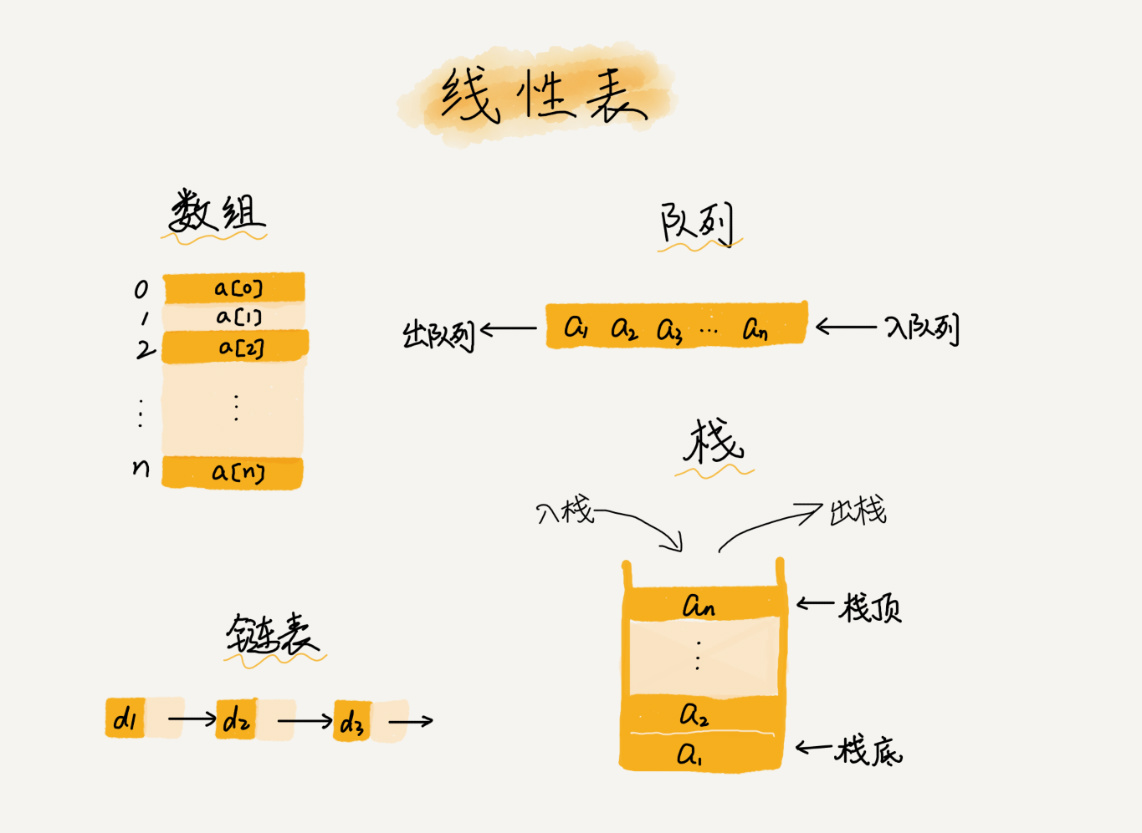1.  数组（Array）是一种线性表数据结构。它用一组连续的内存空间，来存储一组具有相同类型的数据
2.  连续的内存空间和相同类型的数据(随机访问的前提)。
3. 优点：两限制使得具有随机访问的特性缺点：删除，插入数据效率低。
4. 对内存空间要求高，需要一块连续的内存空间。
• 数组怎么根据下标随机访问的？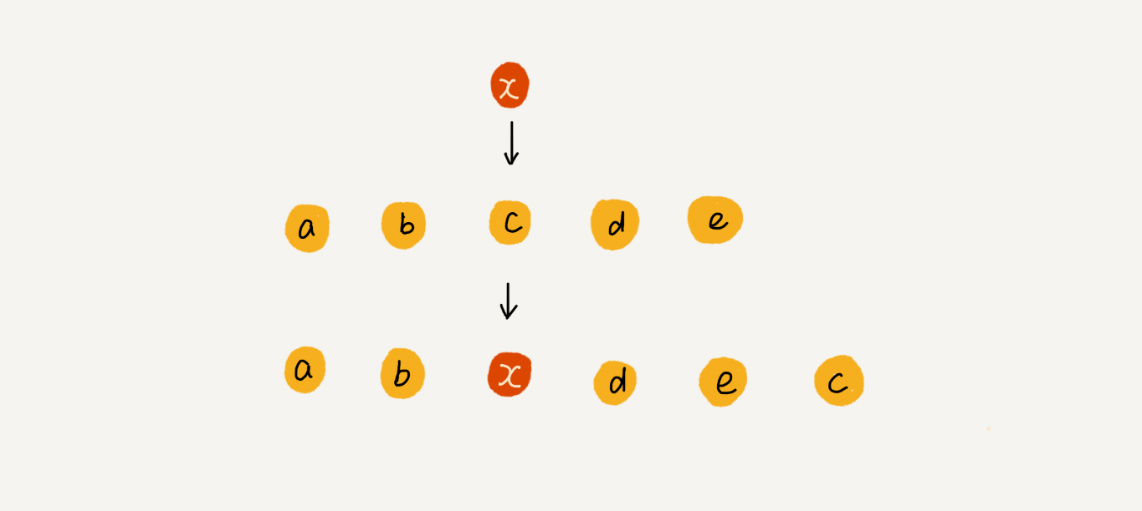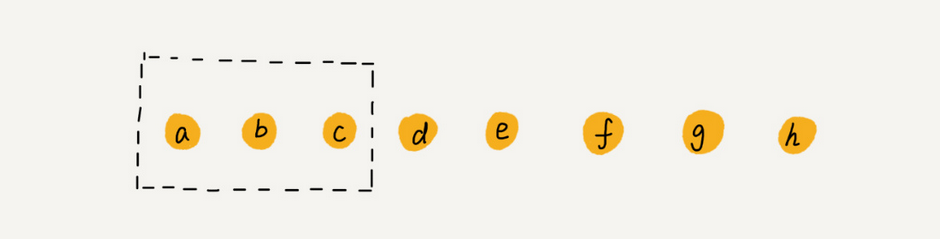2. 低效的插入和删除
1） 插入：从最好O(1) 最坏O(n) 平均O(n)
2） 插入：数组若无序，插入新的元素时，可以将第K个位置元素移动到数组末尾，把心的元素，插入到第k个位置，此处复杂度为O(1)。
3） 删除：从最好O(1) 最坏O(n) 平均O(n)
4） 多次删除集中在一起，提高删除效率

### 2、链表

• 什么是链表

1.和数组一样，链表也是一种线性表。
2.从内存结构来看，链表的内存结构是不连续的内存空间，是将一组零散的内存块串联起来，从而进行数据存储的数据结构。
3.链表中的每一个内存块被称为节点Node。节点除了存储数据外，还需记录链上下一个节点的地址，即后继指针next。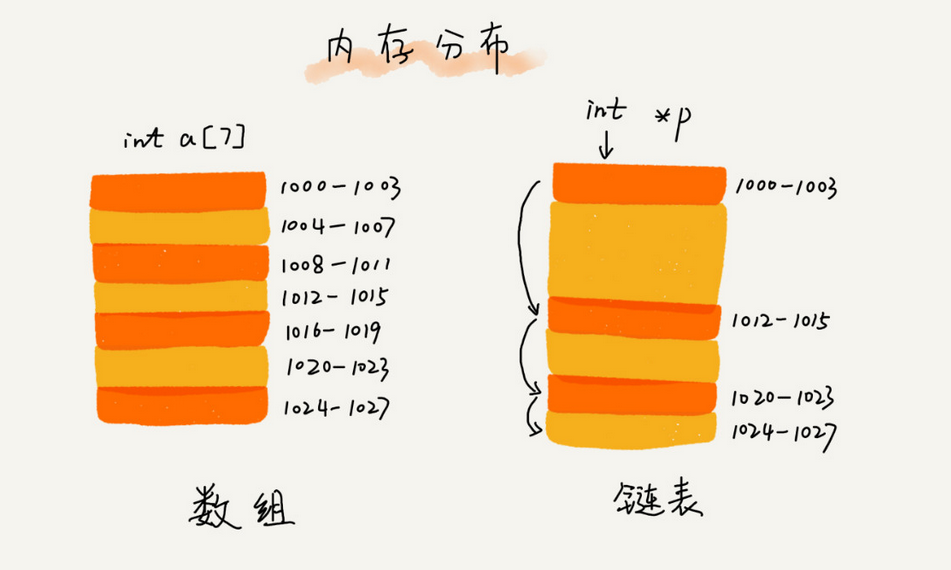• 链表的特点

1.插入、删除数据效率高O(1)级别（只需更改指针指向即可），随机访问效率低O(n)级别（需要从链头至链尾进行遍历）。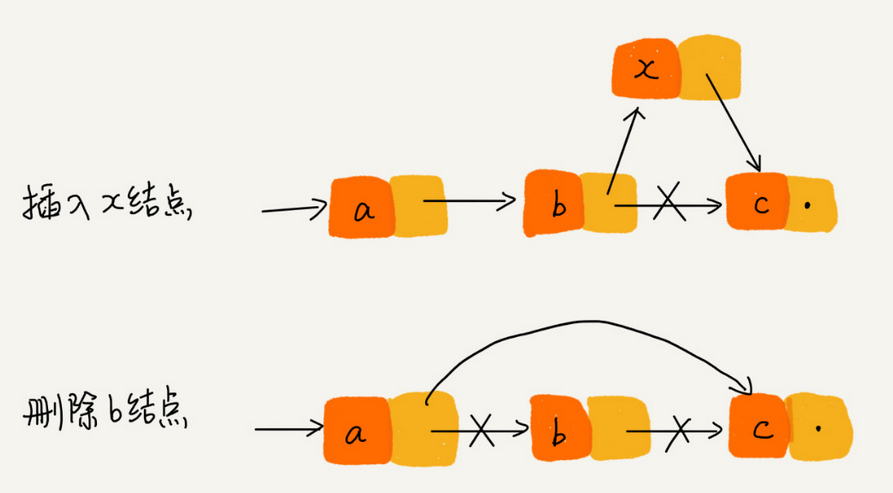2.和数组相比，内存空间消耗更大，因为每个存储数据的节点都需要额外的空间存储后继指针。

• 常用链表

1.单链表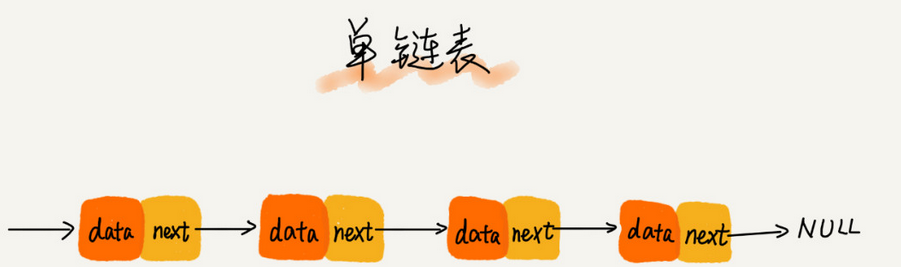1）每个节点只包含一个指针，即后继指针。
2）单链表有两个特殊的节点，即首节点和尾节点。为什么特殊？用首节点地址表示整条链表，尾节点的后继指针指向空地址null。
3）性能特点：插入和删除节点的时间复杂度为O（1），查找的时间复杂度为O(n)。

2.循环链表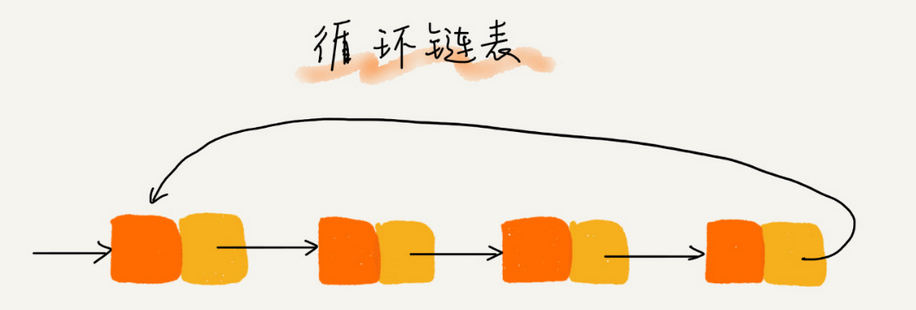1）除了尾节点的后继指针指向首节点的地址外均与单链表一致。
2）适用于存储有循环特点的数据，比如约瑟夫问题。

3.双向链表1）节点除了存储数据外，还有两个指针分别指向前一个节点地址（前驱指针prev）和下一个节点地址（后继指针next）。
2）首节点的前驱指针prev和尾节点的后继指针均指向空地址。
3）性能特点：

4.双向循环链表：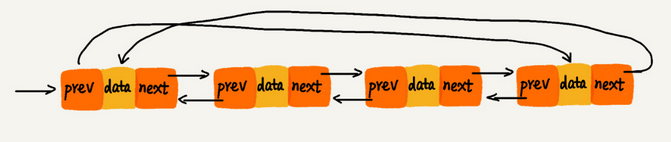• 选择数组还是链表？

1.插入、删除和随机访问的时间复杂度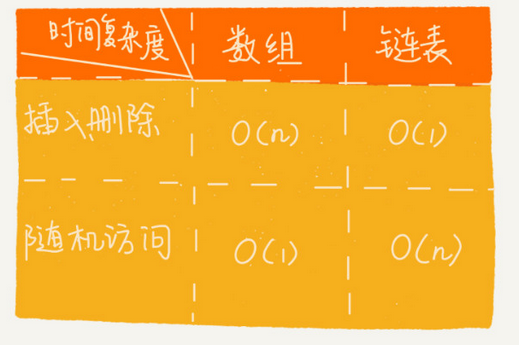2.数组缺点
1）若申请内存空间很大，比如100M，但若内存空间没有100M的连续空间时，则会申请失败，尽管内存可用空间超过100M。
2）大小固定，若存储空间不足，需进行扩容，一旦扩容就要进行数据复制，而这时非常费时的。
3.链表缺点
1）内存空间消耗更大，因为需要额外的空间存储指针信息。
2）对链表进行频繁的插入和删除操作，会导致频繁的内存申请和释放，容易造成内存碎片，如果是Java语言，还可能会造成频繁的GC（自动垃圾回收器）操作。
4.如何选择？

• 应用

1.如何分别用链表和数组实现LRU缓冲淘汰策略？
1）什么是缓存？

2）为什么使用缓存？即缓存的特点

3）什么是缓存淘汰策略？

4）有哪些缓存淘汰策略？

5）链表实现LRU缓存淘汰策略

6）数组实现LRU缓存淘汰策略

2.如何通过单链表实现“判断某个字符串是否为水仙花字符串”？（比如 上海自来水来自海上）
1）前提：字符串以单个字符的形式存储在单链表中。
2）遍历链表，判断字符个数是否为奇数，若为偶数，则不是。
3）将链表中的字符倒序存储一份在另一个链表中。
4）同步遍历2个链表，比较对应的字符是否相等，若相等，则是水仙花字串，否则，不是。

### 3、队列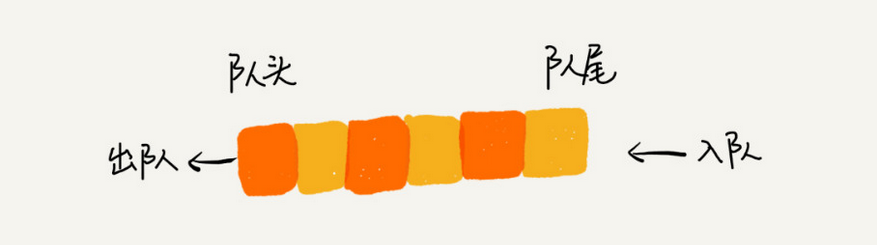1 . 队列跟栈一样,也是一种抽象的数据结构。

2. 具有先进先出的特性,支持在队尾插入元素,在队头删除元素。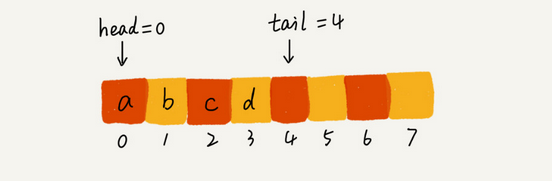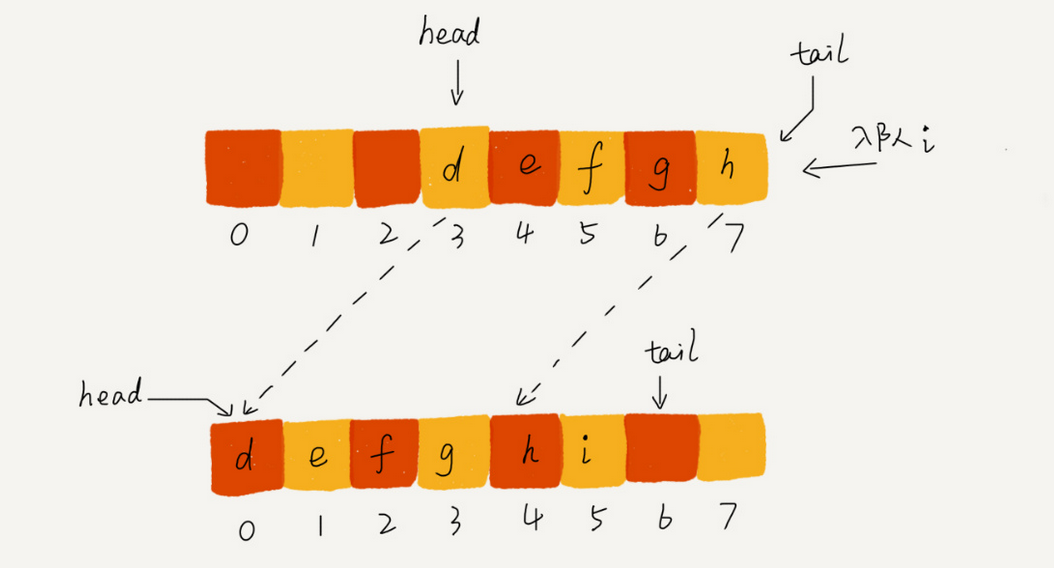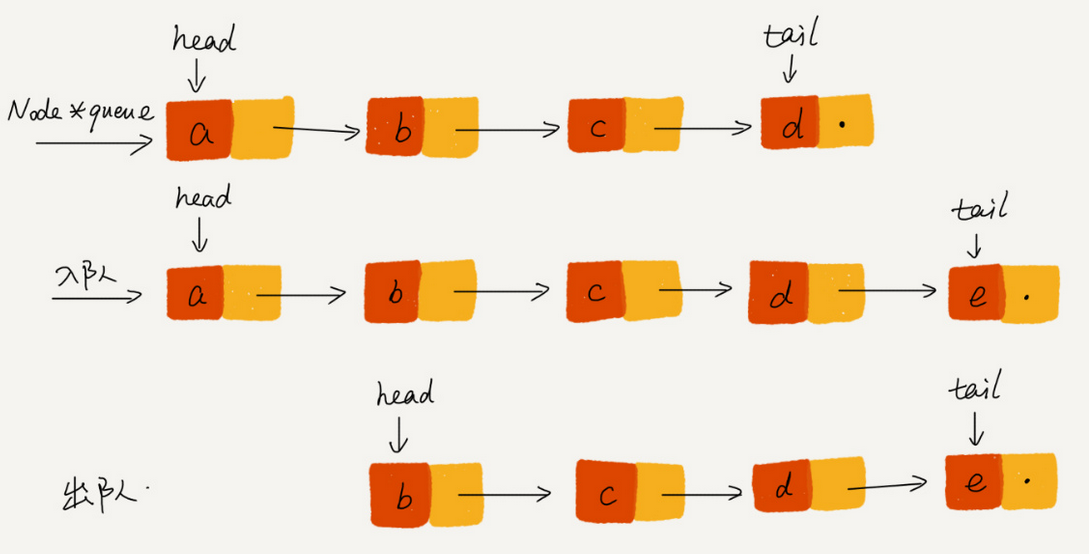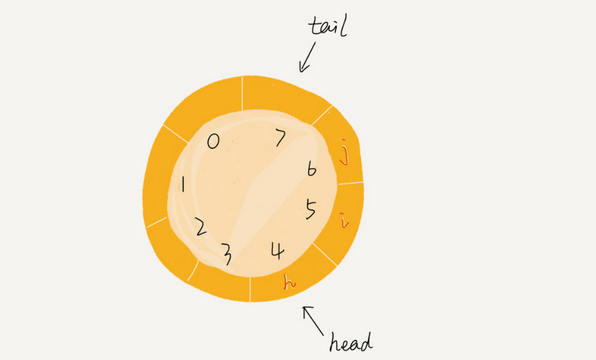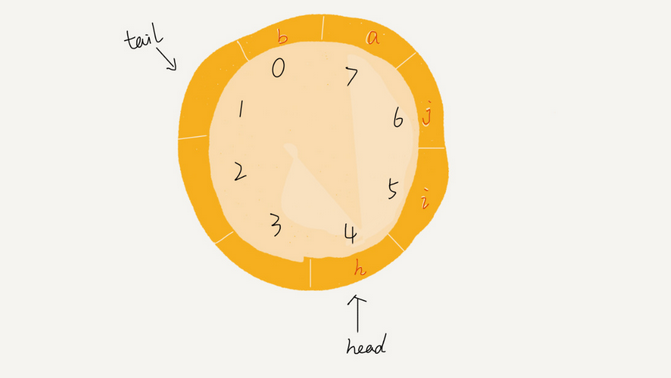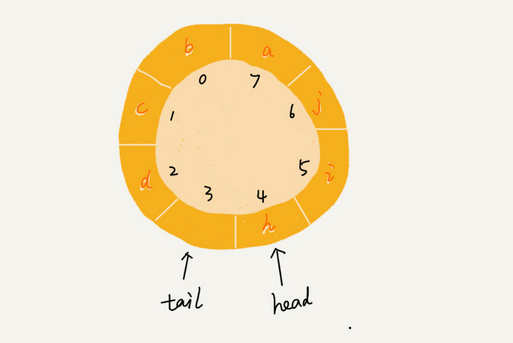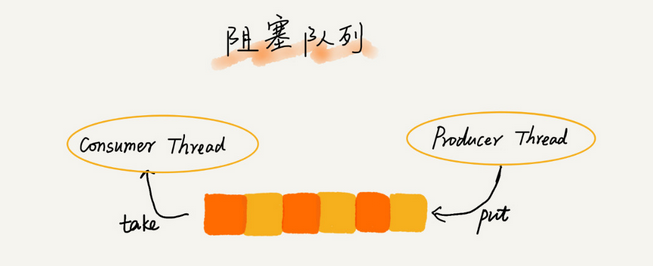### 4、递归算法

1.递归是一种非常高效、简洁的编码技巧，一种应用非常广泛的算法，比如DFS深度优先搜索、前中后序二叉树遍历等都是使用递归。
2.方法或函数调用自身的方式称为递归调用，调用称为递，返回称为归。
3.基本上，所有的递归问题都可以用递推公式来表示，比如
f(n) = f(n-1) + 1;
f(n) = f(n-1) + f(n-2);
f(n)=n*f(n-1);

1.优点：代码的表达力很强，写起来简洁。
2.缺点：空间复杂度高、有堆栈溢出风险、存在重复计算、过多的函数调用会耗时较多等问题。

1.问题的解可以分解为几个子问题的解。何为子问题？就是数据规模更小的问题。
2.问题与子问题，除了数据规模不同，求解思路完全一样
3.存在递归终止条件

1.递归代码编写

2.递归代码理解

1.警惕堆栈溢出：可以声明一个全局变量来控制递归的深度，从而避免堆栈溢出。
2.警惕重复计算：通过某种数据结构来保存已经求解过的值，从而避免重复计算。

### 5、排序

（1）几种最经典、最常用的排序方法：冒泡排序、插入排序、选择排序、快速排序、归并排序、计数排序、基数排序、桶排序。
（2）复杂度归类

<1>算法的执行效率
1. 最好、最坏、平均情况时间复杂度。
2. 时间复杂度的系数、常数和低阶。
3. 比较次数，交换（或移动）次数。
<2>排序算法的稳定性
1. 稳定性概念：如果待排序的序列中存在值相等的元素，经过排序之后，相等元素之间原有的先后顺序不变。
2. 稳定性重要性：可针对对象的多种属性进行有优先级的排序。
3. 举例：给电商交易系统中的“订单”排序，按照金额大小对订单数据排序，对于相同金额的订单以下单时间早晚排序。用稳定排序算法可简洁地解决。先按照下单时间给订单排序，排序完成后用稳定排序算法按照订单金额重新排序。
<3>排序算法的内存损耗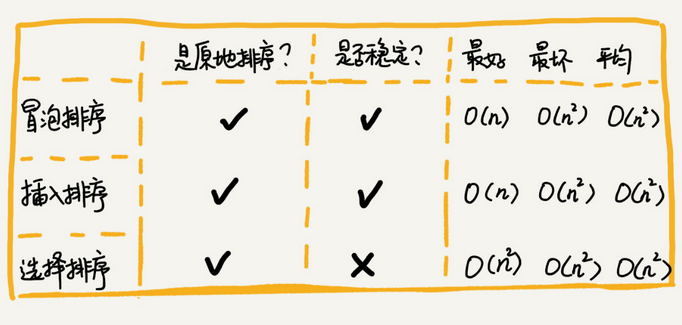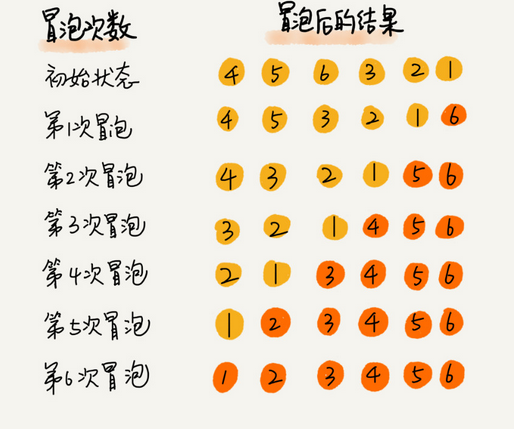public int[] bubbleSort(int[] a) {
int n = a.length;
if (n<=1) {
return a;
}
for (int i = 0; i < n; i++) {
//提前退出冒泡循环的标志
boolean flag = false;
for (int j = 0; j < n-i-1; j++) {
if (a[j]>a[j+1]) {//
int temp = a[j];
a[j] = a[j+1];
a[j+1] = temp;

flag = true;//表示有数据交换
}
if (!flag) {
break; //没有数据交换(说明已排好序无需再进行冒泡),提前退出
}
}
}
return a;
}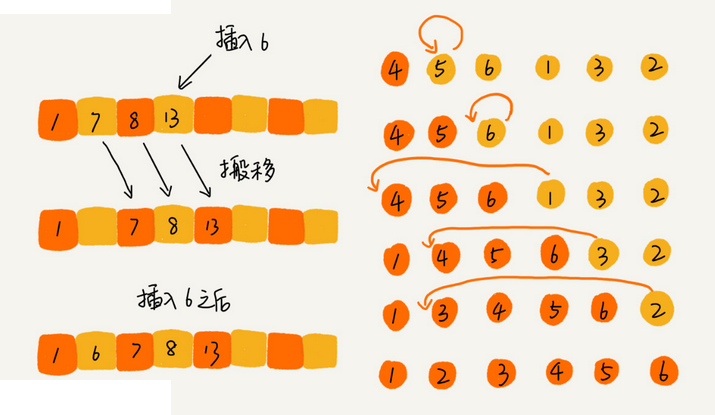public int[] insertionSort(int[] a) {
int n = a.length;
if (n<=1) return a;

for (int i = 1; i < n; i++) {
int value = a[i];
int j = i-1;
for (; j >=0; j--) {
if (a[j] > value) {
a[j+1] = a[j];//移动数据
}else {
break;
}
}
a[j+1] = value;//插入数据
}

return a;
}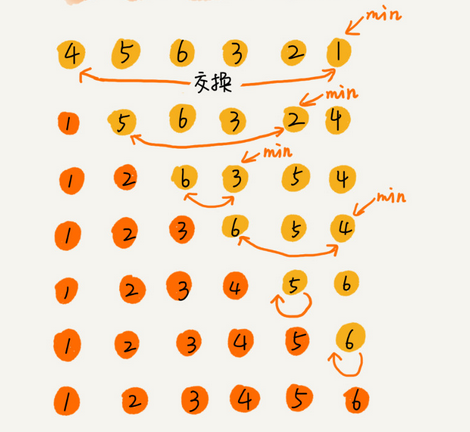public int[] selectionSort(int[] a) {
int n = a.length;

for (int i = 0; i < a.length - 1; i++) {
for (int j = i+1; j < a.length; j++) {
//交换
if (a[i] > a[j]) {
int temp = a[i];
a[i] = a[j];
a[j] = temp;
}
}
}

return a;
}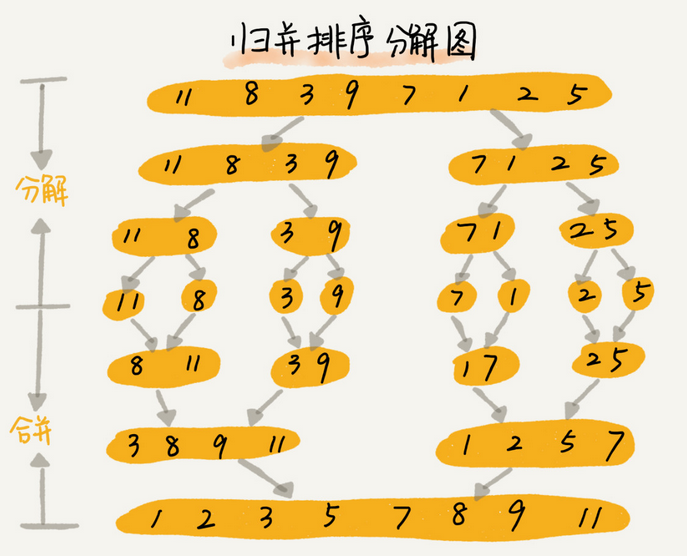实现思路: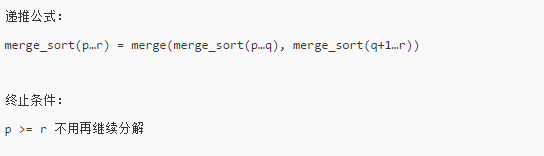merge-sort(p...r)表示,给下标从p到r之间的数组排序。我们将这个排序问题转化为了两个子问 ,题, merge_sort(p...q)和merge-sort(q+1..r),其中下标q等于p和r的中间位置,也就是, (p+r)/2,当下标从p到q和从q+1到r这两个子数组都排好序之后,我们再将两个有序的子数组合并在一起,这样下标从p到r之间的数据就也排好序了。

 // 归并排序算法, a是数组，n表示数组大小
public static void mergeSort(int[] a, int n) {
mergeSortInternally(a, 0, n-1);
}

// 递归调用函数
private static void mergeSortInternally(int[] a, int p, int r) {
// 递归终止条件
if (p >= r) return;

// 取p到r之间的中间位置q
int q = (p+r)/2;
// 分治递归
mergeSortInternally(a, p, q);
mergeSortInternally(a, q+1, r);

// 将A[p...q]和A[q+1...r]合并为A[p...r]
merge(a, p, q, r);
}

private static void merge(int[] a, int p, int q, int r) {
int i = p;
int j = q+1;
int k = 0; // 初始化变量i, j, k
int[] tmp = new int[r-p+1]; // 申请一个大小跟a[p...r]一样的临时数组

// 1 排序
while (i<=q && j<=r) {
if (a[i] <= a[j]) {
tmp[k++] = a[i++]; // i++等于i:=i+1
} else {
tmp[k++] = a[j++];
}
}

// 2 判断哪个子数组中有剩余的数据
int start = i;
int end = q;
if (j <= r) {
start = j;
end = r;
}

// 3 将剩余的数据拷贝到临时数组tmp
while (start <= end) {
tmp[k++] = a[start++];
}

// 4 将tmp中的数组拷贝回a[p...r]
for (i = 0; i <= r-p; ++i) {
a[p+i] = tmp[i];
}
}


merge是这样执行的: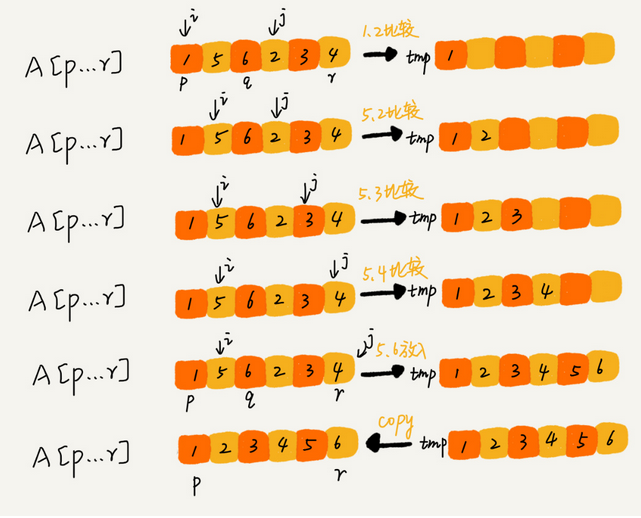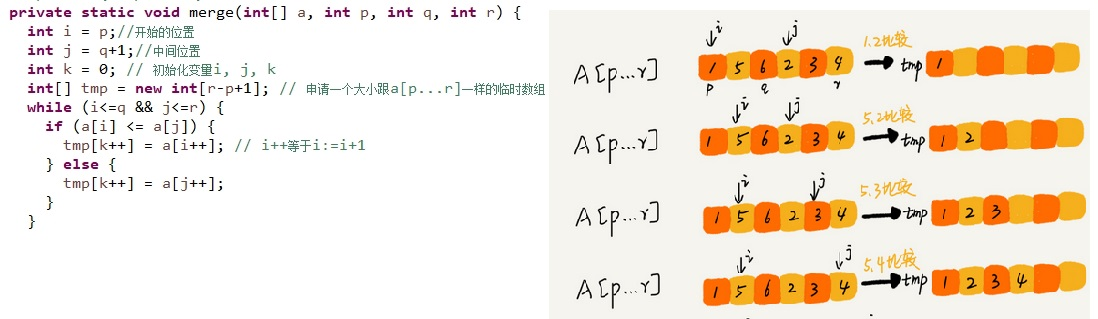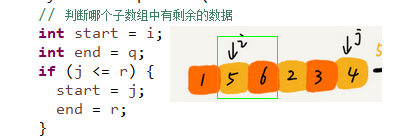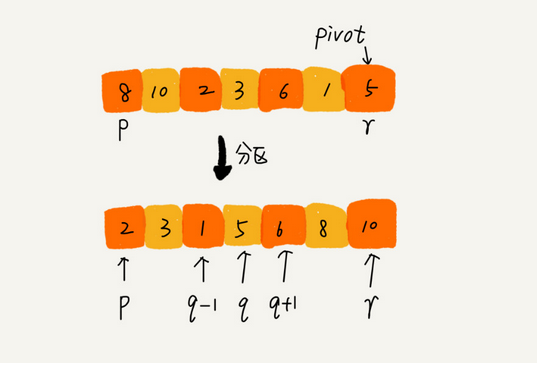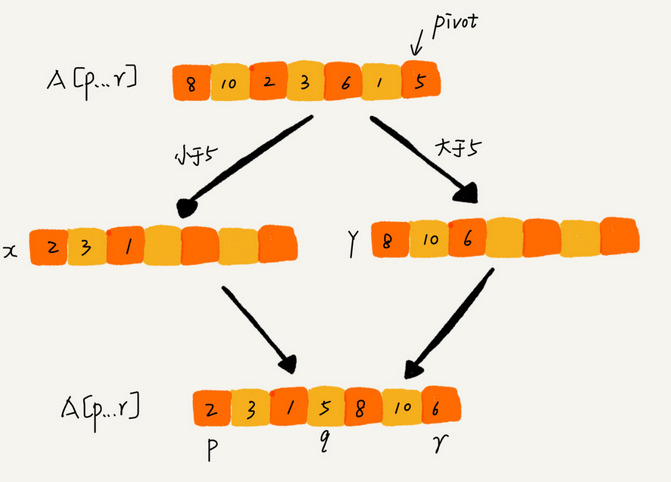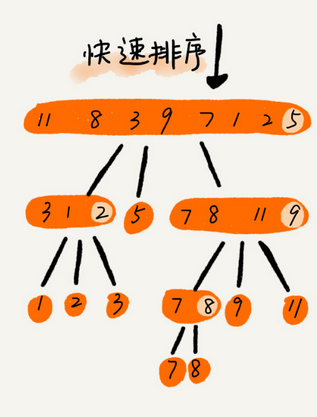#### 八、线性排序:

1, 要排序的数据需要很容易就能划分成m个桶,并且,桶与桶之间有着天然的大小顺序。

2 ,数据在各个桶之间的分布是比较均匀的。

3 ,桶排序比较适合用在外部排序中。所谓的外部排序就是数据存储在外部磁盘中,数据量比较大,内存有限，无法将数据全部加载到内存中。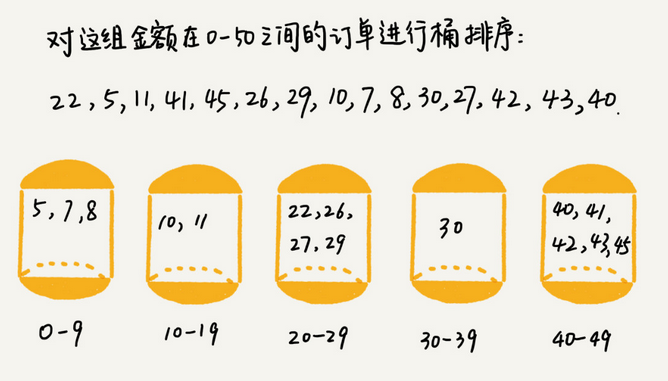// 计数排序，a是数组，n是数组大小。假设数组中存储的都是非负整数。
public static void countingSort(int[] a) {
int n = a.length;
if (n <= 1) return;

// 查找数组中数据的范围
int max = a;
for (int i = 1; i < n; ++i) {
if (max < a[i]) {
max = a[i];
}
}

// 申请一个计数数组c，下标大小[0,max]
int[] c = new int[max + 1];
for (int i = 0; i < max + 1; ++i) {
c[i] = 0;
}

// 计算每个元素的个数，放入c中
for (int i = 0; i < n; ++i) {
c[a[i]]++;
}

// 依次累加
for (int i = 1; i < max + 1; ++i) {
c[i] = c[i-1] + c[i];
}

// 临时数组r，存储排序之后的结果
int[] r = new int[n];
// 计算排序的关键步骤了，有点难理解
for (int i = n - 1; i >= 0; --i) {
int index = c[a[i]]-1;
r[index] = a[i];
c[a[i]]--;
}

// 将结果拷贝会a数组
for (int i = 0; i < n; ++i) {
a[i] = r[i];
}
}

## 散列表

#### 散列函数的设计要求:

1. 散列函数计算得到的散列值是一个非负整数;.
2. 如果key1 = key2,那hash(key1) == hash(key2);
3. 如果key1 != key2,那hash(key1)  !=  hash(key2),

#### 解决散列冲突的方法有两种: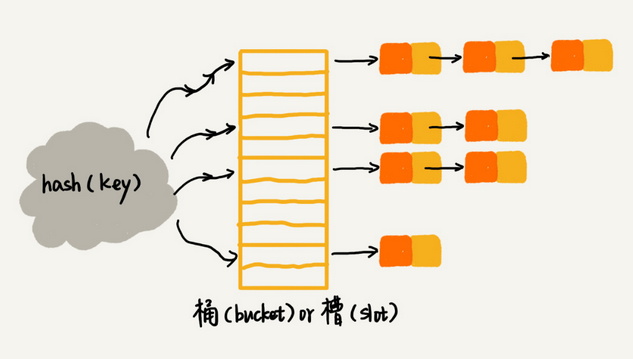04-11
11-28
06-041万+
05-03
04-21
09-15
06-169万+
11-23
08-151万+
11-22
07-212483
10-122156
12-10
01-101962
12-0625万+
12-142898
10-29

### “相关推荐”对你有帮助么？

•非常没帮助
•没帮助
•一般
•有帮助
•非常有帮助被折叠的  条评论 为什么被折叠?到【灌水乐园】发言¥1 ¥2 ¥4 ¥6 ¥10 ¥20获取中扫码支付点击重新获取扫码支付1.余额是钱包充值的虚拟货币，按照1:1的比例进行支付金额的抵扣。
2.余额无法直接购买下载，可以购买VIP、付费专栏及课程。余额充值